### Home > CALC > Chapter 5 > Lesson 5.1.2 > Problem5-14

5-14.
1.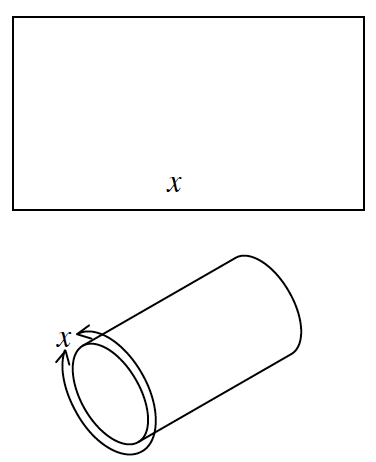A rectangular piece of sheet metal with a perimeter of 50 cm is rolled into a cylinder with two open ends. Homework Help ✎

1. Find the radius and height of the cylinder in terms of x.

2. Express the volume of the cylinder as a function of x.

3. Find the value of x that will maximize the volume and find the maximum value.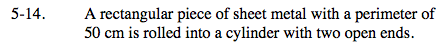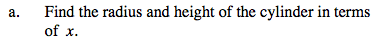Look at the diagram of the cylinder. The circumference of the base is equivalent to x.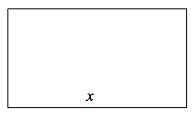P = 2b + 2h
25 = 2x+2h
Solve for h.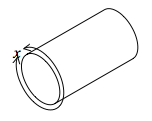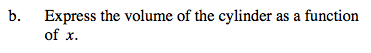V = π(r²)(h). Substitute.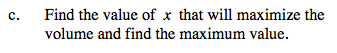The volume will be at a maximum when its derivative is 0.